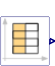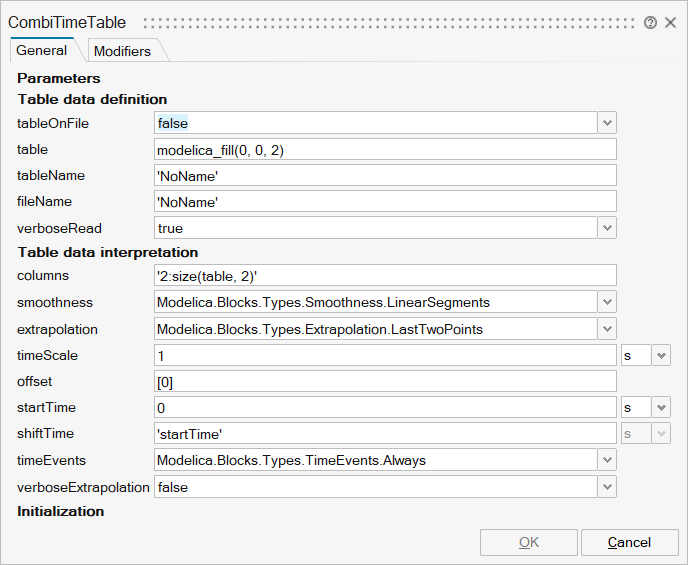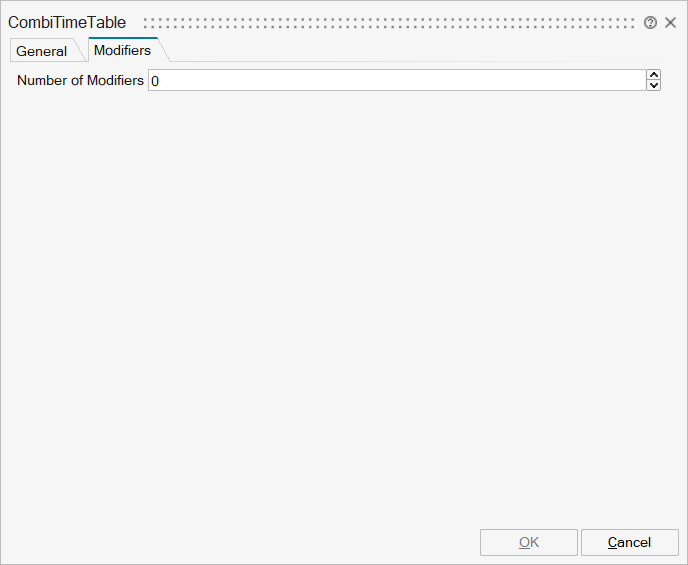# CombiTimeTable

Table look-up with respect to time and linear/periodic extrapolation methods (data from matrix/file)## Library

Modelica/Blocks/Sources

## Description

This block generates an output signal y[:] by constant, linear or cubic Hermite spline interpolation in a table. The time points and function values are stored in a matrix table[i,j], where the first column table[:,1] contains the time points and the other columns contain the data to be interpolated.Via parameter columns it can be defined which columns of the table are interpolated. If, e.g., columns={2,4}, it is assumed that 2 output signals are present and that the first output is computed by interpolation of column 2 and the second output is computed by interpolation of column 4 of the table matrix. The table interpolation has the following properties:

• The interpolation interval is found by a binary search where the interval used in the last call is used as start interval.
• The time points need to be strictly increasing for cubic Hermite spline interpolation, otherwise monotonically increasing.
• Discontinuities are allowed for (constant or) linear interpolation, by providing the same time point twice in the table.
• Via parameter smoothness it is defined how the data is interpolated:
  smoothness = 1: Linear interpolation
= 2: Akima interpolation: Smooth interpolation by cubic Hermite
splines such that der(y) is continuous, also if extrapolated.
= 3: Constant segments
= 4: Fritsch-Butland interpolation: Smooth interpolation by cubic
Hermite splines such that y preserves the monotonicity and
der(y) is continuous, also if extrapolated.
= 5: Steffen interpolation: Smooth interpolation by cubic Hermite
splines such that y preserves the monotonicity and der(y)
is continuous, also if extrapolated.

• Values outside of the table range, are computed by extrapolation according to the setting of parameter extrapolation:
  extrapolation = 1: Hold the first or last value of the table,
if outside of the table scope.
= 2: Extrapolate by using the derivative at the first/last table
points if outside of the table scope.
(If smoothness is LinearSegments or ConstantSegments
this means to extrapolate linearly through the first/last
two table points.).
= 3: Periodically repeat the table data (periodical function).
= 4: No extrapolation, i.e. extrapolation triggers an error

• If the table has only one row, no interpolation is performed and the table values of this row are just returned.
• Via parameters shiftTime and offset the curve defined by the table can be shifted both in time and in the ordinate value. The time instants stored in the table are therefore relative to shiftTime.
• If time < startTime, no interpolation is performed and the offset is used as ordinate value for all outputs.
• The table is implemented in a numerically sound way by generating time events at interval boundaries, in case of interpolation by linear segments. This generates continuously differentiable values for the integrator. Via parameter timeEvents it is defined how the time events are generated:
  timeEvents = 1: Always generate time events at interval boundaries
= 2: Generate time events at discontinuities (defined by duplicated sample points)
= 3: No time events at interval boundaries

For interpolation by constant segments time events are always generated at interval boundaries. For smooth interpolation by cubic Hermite splines no time events are generated at interval boundaries.
• Via parameter timeScale the first column of the table array can be scaled, e.g., if the table array is given in hours (instead of seconds) timeScale shall be set to 3600.
• For special applications it is sometimes needed to know the minimum and maximum time instant defined in the table as a parameter. For this reason parameters t_min/t_minScaled and t_max/t_maxScaled are provided and can be accessed from the outside of the table object. Whereas t_min and t_max define the scaled abscissa values (using parameter timeScale) in SIunits.Time, t_minScaled and t_maxScaled define the unitless original abscissa values of the table.

Example:

   table = [0, 0;
1, 0;
1, 1;
2, 4;
3, 9;
4, 16];
extrapolation = 2 (default), timeEvents = 2
If, e.g., time = 1.0, the output y =  0.0 (before event), 1.0 (after event)
e.g., time = 1.5, the output y =  2.5,
e.g., time = 2.0, the output y =  4.0,
e.g., time = 5.0, the output y = 23.0 (i.e., extrapolation via last 2 points).


The table matrix can be defined in the following ways:

1. Explicitly supplied as parameter matrix "table", and the other parameters have the following values:
   tableName is "NoName" or has only blanks,
fileName  is "NoName" or has only blanks.

2. Read from a file "fileName" where the matrix is stored as "tableName". Both text and MATLAB MAT-file format is possible. (The text format is described below). The MAT-file format comes in four different versions: v4, v6, v7 and v7.3. The library supports at least v4, v6 and v7 whereas v7.3 is optional. It is most convenient to generate the MAT-file from FreeMat or MATLAB® by command
   save tables.mat tab1 tab2 tab3

or Scilab by command
   savematfile tables.mat tab1 tab2 tab3

when the three tables tab1, tab2, tab3 should be used from the model.
Note, a fileName can be defined as URI by using the helper function loadResource.
3. Statically stored in function "usertab" in file "usertab.c". The matrix is identified by "tableName". Parameter fileName = "NoName" or has only blanks. Row-wise storage is always to be preferred as otherwise the table is reallocated and transposed.

When the constant "NO_FILE_SYSTEM" is defined, all file I/O related parts of the source code are removed by the C-preprocessor, such that no access to files takes place.

If tables are read from a text file, the file needs to have the following structure ("-----" is not part of the file content):

-----------------------------------------------------
#1
double tab1(6,2)   # comment line
0   0
1   0
1   1
2   4
3   9
4  16
double tab2(6,2)   # another comment line
0   0
2   0
2   2
4   8
6  18
8  32
-----------------------------------------------------


Note, that the first two characters in the file need to be "#1" (a line comment defining the version number of the file format). Afterwards, the corresponding matrix has to be declared with type (= "double" or "float"), name and actual dimensions. Finally, in successive rows of the file, the elements of the matrix have to be given. The elements have to be provided as a sequence of numbers in row-wise order (therefore a matrix row can span several lines in the file and need not start at the beginning of a line). Numbers have to be given according to C syntax (such as 2.3, -2, +2.e4). Number separators are spaces, tab (\\t), comma (,), or semicolon (;). Several matrices may be defined one after another. Line comments start with the hash symbol (#) and can appear everywhere. Text files should either be ASCII or UTF-8 encoded, where UTF-8 encoded strings are only allowed in line comments and an optional UTF-8 BOM at the start of the text file is ignored. Other characters, like trailing non comments, are not allowed in the file.

MATLAB is a registered trademark of The MathWorks, Inc.

## ParametersNameLabelDescriptionData TypeValid Values

mo_nout

nout

Number of outputs

Scalar

mo_tableOnFile

tableOnFile

= true, if table is defined on file or in function usertab

Scalar

true
false

mo_table

table

Table matrix (time = first column; e.g., table=[0, 0; 1, 1; 2, 4])

Matrix

mo_tableName

tableName

Table name on file or in function usertab (see docu)

String

mo_fileName

fileName

File where matrix is stored

String

= true, if info message that file is loading is to be printed

Scalar

true
false

mo_columns

columns

Columns of table to be interpolated

Vector

mo_smoothness

smoothness

Smoothness of table interpolation

Structure

mo_smoothness/choice1

Table points are linearly interpolated

Number

0
1

mo_smoothness/choice2

Table points are interpolated (by Akima splines) such that the first derivative is continuous

Number

0
1

mo_smoothness/choice3

Table points are not interpolated, but the value from the previous abscissa point is returned

Number

0
1

mo_smoothness/choice4

Table points are interpolated (by Fritsch-Butland splines) such that the monotonicity is preserved and the first derivative is continuous

Number

0
1

mo_smoothness/choice5

Table points are interpolated (by Steffen splines) such that the monotonicity is preserved and the first derivative is continuous

Number

0
1

mo_extrapolation

extrapolation

Extrapolation of data outside the definition range

Structure

mo_extrapolation/choice1

Hold the first/last table point outside of the table scope

Number

0
1

mo_extrapolation/choice2

Extrapolate by using the derivative at the first/last table points outside of the table scope

Number

0
1

mo_extrapolation/choice3

Repeat the table scope periodically

Number

0
1

mo_extrapolation/choice4

Extrapolation triggers an error

Number

0
1

mo_timeScale

timeScale

Time scale of first table column

Scalar

mo_offset

offset

Offsets of output signals

Vector

mo_startTime

startTime

Output = offset for time < startTime

Scalar

mo_shiftTime

shiftTime

Shift time of first table column

Scalar

mo_timeEvents

timeEvents

Time event handling of table interpolation

Structure

mo_timeEvents/choice1

Always generate time events at interval boundaries

Number

0
1

mo_timeEvents/choice2

Generate time events at discontinuities (defined by duplicated sample points)

Number

0
1

mo_timeEvents/choice3

No time events at interval boundaries

Number

0
1

mo_verboseExtrapolation

verboseExtrapolation

= true, if warning messages are to be printed if time is outside the table definition range

Scalar

true
false

mo_t_min

t_min

Minimum abscissa value defined in table

Scalar

mo_t_max

t_max

Maximum abscissa value defined in table

Scalar

mo_t_minScaled

t_minScaled

Minimum (scaled) abscissa value defined in table

Scalar

mo_t_maxScaled

t_maxScaled

Maximum (scaled) abscissa value defined in table

Scalar

mo_p_offset

p_offset

Offsets of output signals

Vector

mo_tableID

tableID

External table object

ScalarNameLabelDescriptionData TypeValid Values

mo_y

y

y

Structure

mo_y/fixed

fixed

Cell of vectors

true
false

mo_y/start

start

Cell of vectors

## Ports

NameTypeDescriptionIO TypeNumber

y

implicit

Connector of Real output signals

output

1